Coefficient of couplingCustom SearchCOEFFICIENT OF COUPLING The COEFFICIENT OF COUPLING of a transformer is dependent on the portion of the total flux lines that cuts both primary and secondary windings. Ideally, all the flux lines generated by the primary should cut the secondary, and all the lines of the flux generated by the secondary should cut the primary. The coefficient of coupling would then be one (unity), and maximum energy would be transferred from the primary to the secondary. Practical power transformers use high-permeability silicon steel cores and close spacing between the windings to provide a high coefficient of coupling. Lines of flux generated by one winding which do not link with the other winding are called LEAKAGE FLUX. Since leakage flux generated by the primary does not cut the secondary, it cannot induce a voltage into the secondary. The voltage induced into the secondary is therefore less than it would be if the leakage flux did not exist. Since the effect of leakage flux is to lower the voltage induced into the secondary, the effect can be duplicated by assuming an inductor to be connected in series with the primary. This series LEAKAGE INDUCTANCE is assumed to drop part of the applied voltage, leaving less voltage across the primary. Q.14 What is "leakage flux?" Q.15 What effect does flux leakage in a transformer have on the coefficient of coupling (K) in the transformer? TURNS AND VOLTAGE RATIOS The total voltage induced into the secondary winding of a transformer is determined mainly by the RATIO of the number of turns in the primary to the number of turns in the secondary, and by the amount of voltage applied to the primary. Refer to figure 5-10. Part (A) of the figure shows a transformer whose primary consists of ten turns of wire and whose secondary consists of a single turn of wire. You know that as lines of flux generated by the primary expand and collapse, they cut BOTH the ten turns of the primary and the single turn of the secondary. Since the length of the wire in the secondary is approximately the same as the length of the wire in each turn in the primary, EMF INDUCED INTO THE SECONDARY WILL BE THE SAME AS THE EMF INDUCED INTO EACH TURN IN THE PRIMARY. This means that if the voltage applied to the primary winding is 10 volts, the counter emf in the primary is almost 10 volts. Thus, each turn in the primary will have an induced counter emf of approximately one-tenth of the total applied voltage, or one volt. Since the same flux lines cut the turns in both the secondary and the primary, each turn will have an emf of one volt induced into it. The transformer in part (A) of figure 5-10 has only one turn in the secondary, thus, the emf across the secondary is one volt. Figure 5-10. - Transformer turns and voltage ratios.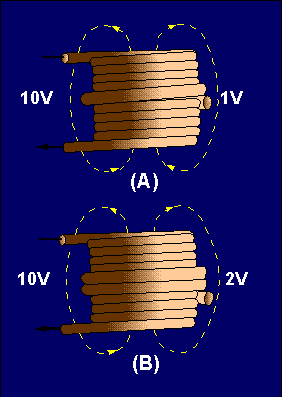The transformer represented in part (B) of figure 5-10 has a ten-turn primary and a two-turn secondary. Since the flux induces one volt per turn, the total voltage across the secondary is two volts. Notice that the volts per turn are the same for both primary and secondary windings. Since the counter emf in the primary is equal (or almost) to the applied voltage, a proportion may be set up to express the value of the voltage induced in terms of the voltage applied to the primary and the number of turns in each winding. This proportion also shows the relationship between the number of turns in each winding and the voltage across each winding. This proportion is expressed by the equation: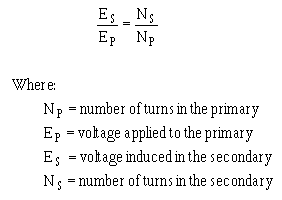Notice the equation shows that the ratio of secondary voltage to primary voltage is equal to the ratio of secondary turns to primary turns. The equation can be written as:The following formulas are derived from the above equation: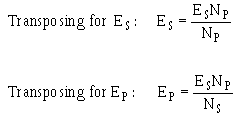If any three of the quantities in the above formulas are known, the fourth quantity can be calculated. Example. A transformer has 200 turns in the primary, 50 turns in the secondary, and 120 volts applied to the primary (Ep). What is the voltage across the secondary (E s)?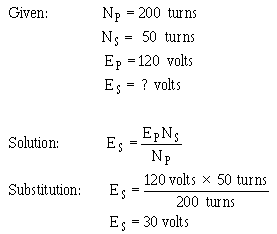Example. There are 400 turns of wire in an iron-core coil. If this coil is to be used as the primary of a transformer, how many turns must be wound on the coil to form the secondary winding of the transformer to have a secondary voltage of one volt if the primary voltage is five volts?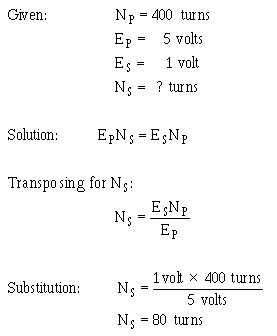Note: The ratio of the voltage (5:1) is equal to the turns ratio (400:80). Sometimes, instead of specific values, you are given a turns or voltage ratio. In this case, you may assume any value for one of the voltages (or turns) and compute the other value from the ratio. For example, if a turn ratio is given as 6:1, you can assume a number of turns for the primary and compute the secondary number of turns (60:10, 36:6, 30:5, etc.). The transformer in each of the above problems has fewer turns in the secondary than in the primary. As a result, there is less voltage across the secondary than across the primary. A transformer in which the voltage across the secondary is less than the voltage across the primary is called a STEP-DOWN transformer. The ratio of a four-to-one step-down transformer is written as 4:1. A transformer that has fewer turns in the primary than in the secondary will produce a greater voltage across the secondary than the voltage applied to the primary. A transformer in which the voltage across the secondary is greater than the voltage applied to the primary is called a STEP-UP transformer. The ratio of a one-to-four step-up transformer should be written as 1:4. Notice in the two ratios that the value of the primary winding is always stated first. Q.16 Does 1:5 indicate a step-up or step-down transformer? Q.17 A transformer has 500 turns on the primary and 1500 turns on the secondary. If 45 volts are applied to the primary, what is the voltage developed across the secondary? (Assume no losses) Q.18 A transformer has a turns ratio of 7:1. If 5 volts is developed across the secondary, what is the voltage applied to the primary? (Note: Es is given, what is Ep?) Q.19 A transformer has 60 volts applied to its primary and 420 volts appearing across its secondary. If there are 800 turns on the primary, what is the number of turns in the secondary?Integrated Publishing, Inc.